## Solution to 1986 Problem 61

 Let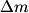$\Delta m$ be the change in the mass of the rocket during a small time interval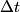$\Delta t$. So,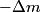$-\Delta m$ is the mass of the exhaust released from the rocket during the time interval$\Delta t$. Let$v$ be the velocity of the rocket before the mass$-\Delta m$ is released,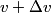$v + \Delta v$ be the velocity of the rocket after the mass$-\Delta m$ is released, and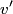$v'$ be the velocity of the mass$-\Delta m$ after it is released. Conservation of momentum tells us that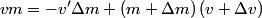\begin{align*}v m = -v' \Delta m + \left(m + \Delta m\right)\left(v + \Delta v \right)\end{align*}Ignoring the second order term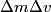$\Delta m \Delta v$ gives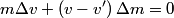\begin{align*}m \Delta v + \left(v - v' \right) \Delta m = 0\end{align*}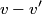$v - v'$ is the speed of the exhaust relative to the rocket, so answer (E) is correct.